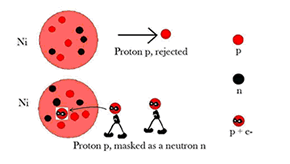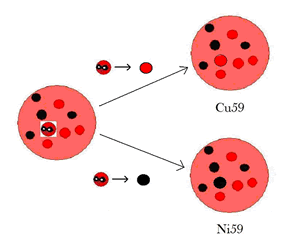# How can 30% of nickel in Rossi’s reactor be transmuted into copper?

by Dott. Giuliano Bettini
Retired. Earlier: Selenia SpA, Rome and IDS SpA, Pisa
Also Adjunct Professor at the University of Pisa

Abstract
In the present article I would like to answer a question posed by L. Kowalsky in a recent paper: how can 30% of nickel in Rossi’s reactor be transmuted into copper? “Everything should be made as simple as possible, but not simpler”, says a guy. I apologizes if I am too simplistic here.

Introduction
The interest on Andrea Rossi’s Nickel-Hydrogen Cold Fusion technology is accelerating . However, Rossi says that about 30% of nickel was turned into copper, after 6 months of uninterrupted operation. Kowalski . says that “this seems to be impossible because the produced copper isotopes rapidly decay into Ni”. But how it works?

How it works
Following Focardi Rossi . a Ni58 nucleus produces a Copper nucleus according to the reaction

Ni58 + p → Cu59

Copper nucleus Cu59 decays with positron (e+) and neutrino (ν) emission in Ni59 nucleus according to

Cu59 → Ni59 + ν + e+

Then (e+) annichilates with (e-) in two gamma-rays

e- + e+ → γ + γ

Starting  from Ni58 which is the more abundant isotope, we can obtain as described in the two above processes Copper formation and its successive decay in Nickel, producing Ni59, Ni60, Ni61 and Ni62. Because Cu63, which can be formed starting by Ni62, is stable and does not decay in Ni63, the chain stops at Ni62 (i.e. Cu63). Each process means some MeV.

Of course how can a proton p gets captured by the Ni58 nucleus? (and subsequent Ni59, Ni60, Ni61 and Ni62). Following Stremmenos . a neutron-like particle, an electron proton pair, a mini-atom, a proton masked as a neutron, gets captured by the Ni58.If the masked proton becomes a neutron the result is Ni59.
In order to have Cu59 (increase of atomic number from 28 to 29) the electron (of the masked proton) gets ejected from the nucleus. The masked proton becomes a proton.The same process holds for all the subsequent transformations, until Cu63.
It remains to be understood the issue of the gamma radiation in the MeV range.

Numbers
I am an electronic engineer, so I need easy numbers in order to understand.
However “Everything should be made as simple as possible, but not simpler”, says a guy. Maybe I am too simple here.
Let’s calculate.

MeV for each Ni transformation
I read that starting from Ni58 we can obtain Copper formation and its successive decay in Nickel, producing Ni59, Ni60, and Ni62. The chain stops at Cu63 stable.
For simplicity I assume all the Nickel in the reactor in the form Ni58.
For simplicity I suppose for each Ni58 the whole sequence of events from Ni58 to Cu63 and as a rough estimate I calculate the mass defect between (Ni58 plus 5 nucleons) and the final state Cu63.
Ni58 mass is calculated to be 57.95380± 15 amu
The actual mass of a copper-Cu63 nucleus is 62.91367 amu
Mass of Ni58 plus 5 nucleons is  57.95380+5=62.95380 amu
Mass defect is 62.95380-62.91367=0.04013 amu
1 amu = 931 MeV is used as a standard conversion
0.04013×931 MeV=37.36 MeV
So each transformation of Ni58 into Cu63 releases 37.36MeV of nuclear energy.

Nickel consumption
According to many blogs in the Internet “One hundred grams of nickel powder can power a 10 kW unit for a minimum of six months”.
How much of Ni58 should be transformed, in six months of continuous operation, in order to generate 10 kW?
I follow a procedure outlined in .
10 kW is thermal or electrical (?) power. The nuclear power must be larger. Assume a nuclear power twice:
20 kW = 20,000 J/s = 1.25 x 10**17 MeV/s.
Each transformation of Ni58 into Cu63 releases 37.36MeV of nuclear energy.
The number of Ni58 transformations should thus be equal to (1.25 x 10**17)/37.36 = 3.346 x 10**15 per second.
Multiplying by the number of seconds in six months (1.55 x 10**7) the total number of transformed Ni58 nuclei is 5.186 x 10**22.
This means 5 grams.
The order of magnitude is not exactly the same but seems to be plausible. This means also 5 grams of Nickel in Rossi’s reactor transmuted into (stable) Copper after six months of continuous operation at the rate of 10 kW.

Conclusions
Rossi says that about 30% of nickel was turned into copper, after 6 months of uninterrupted operation. At first glance this seems to agree with calculations based on simple assumptions.

References

### 1,007 comments to How can 30% of nickel in Rossi’s reactor be transmuted into copper?

• Andrea Rossi

Christian SCHOLL:
Warm Regards,
A.R.

• christian SCHOLL

Dear Andrea Rossi,

A video on alternative energy sources

Best regards,

Christian

• Andrea Rossi

Christian Scholl:
1- It is possible
2- Should be 30
3- It can work also in the total dark, although moonlight is more romantic…
Warm Regards,
A.R.

• Andrea Rossi

Mats Heijkenskjold:
NGU: Never Give Up.
Yes,SKLep NGU is the updated model designed after the failure of the EV test we made.
Dimensions and weight are going to be modified, as you can see also in our website
http://www.ecat.com
Warm Regards,
A.R.

• Mats Heijkenskjold

Dear Andrea,

NGU, what is the meaning of the letters?

The electrical difference between the “old” SKLep and the new NGU? Are they not in principle the same?

Thanks for some clarification!

Best regards

Mats Heijkenskjold

• christian SCHOLL

Hello Andrea Rossi,

Some questions about the E-Cat NGU.
1) Will it be interconnected between solar panels and inverter?
2) E-Cat NGU has a high COP, probably close to 50?
3) In this case, the solar inverter can work at night with moonlight?
4) What is the expected KWH production amplification factor?
Best regards,

Christian

• Andrea Rossi

Christian SCHOLL:
Great !
Warm Regards,
A.R.